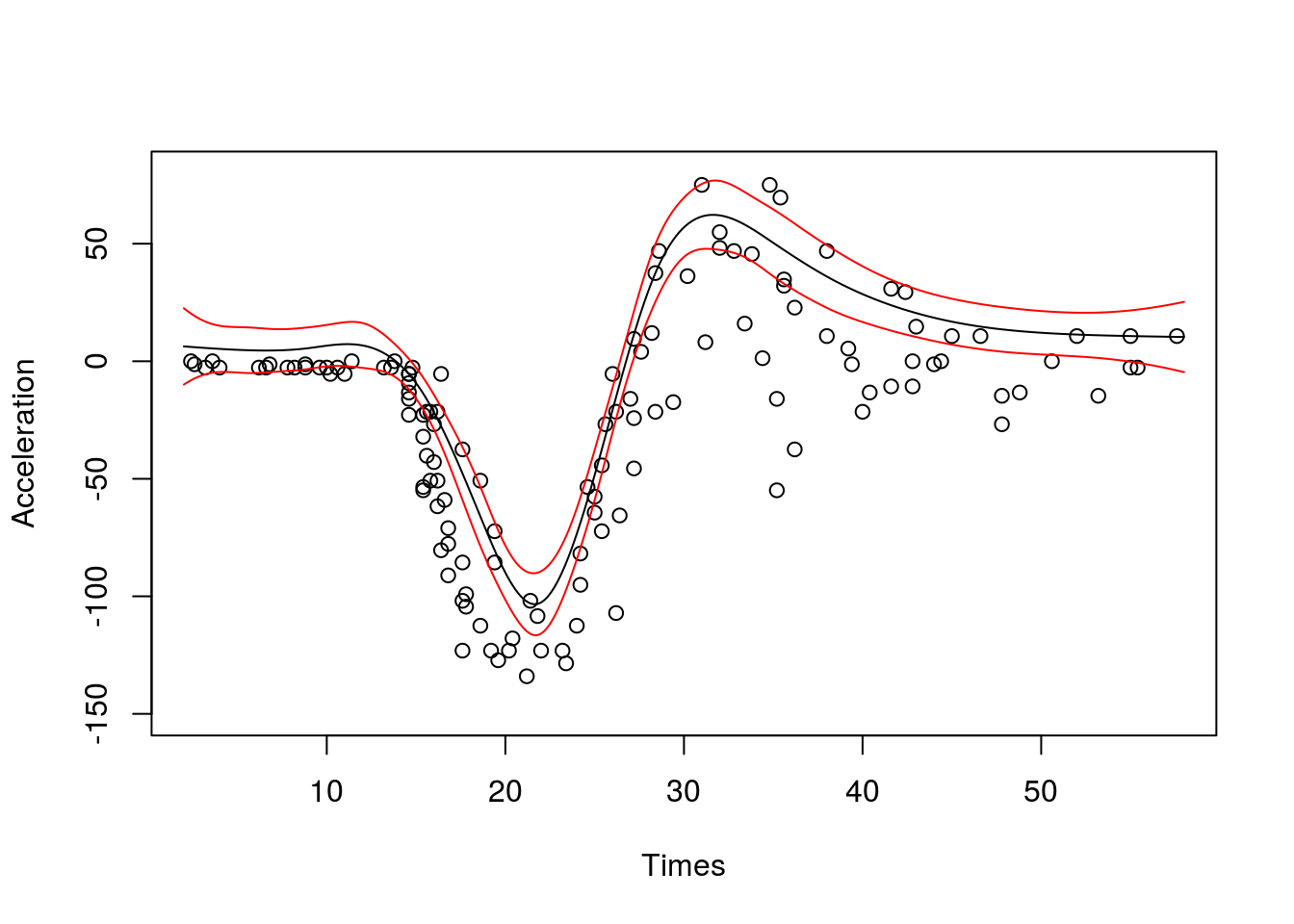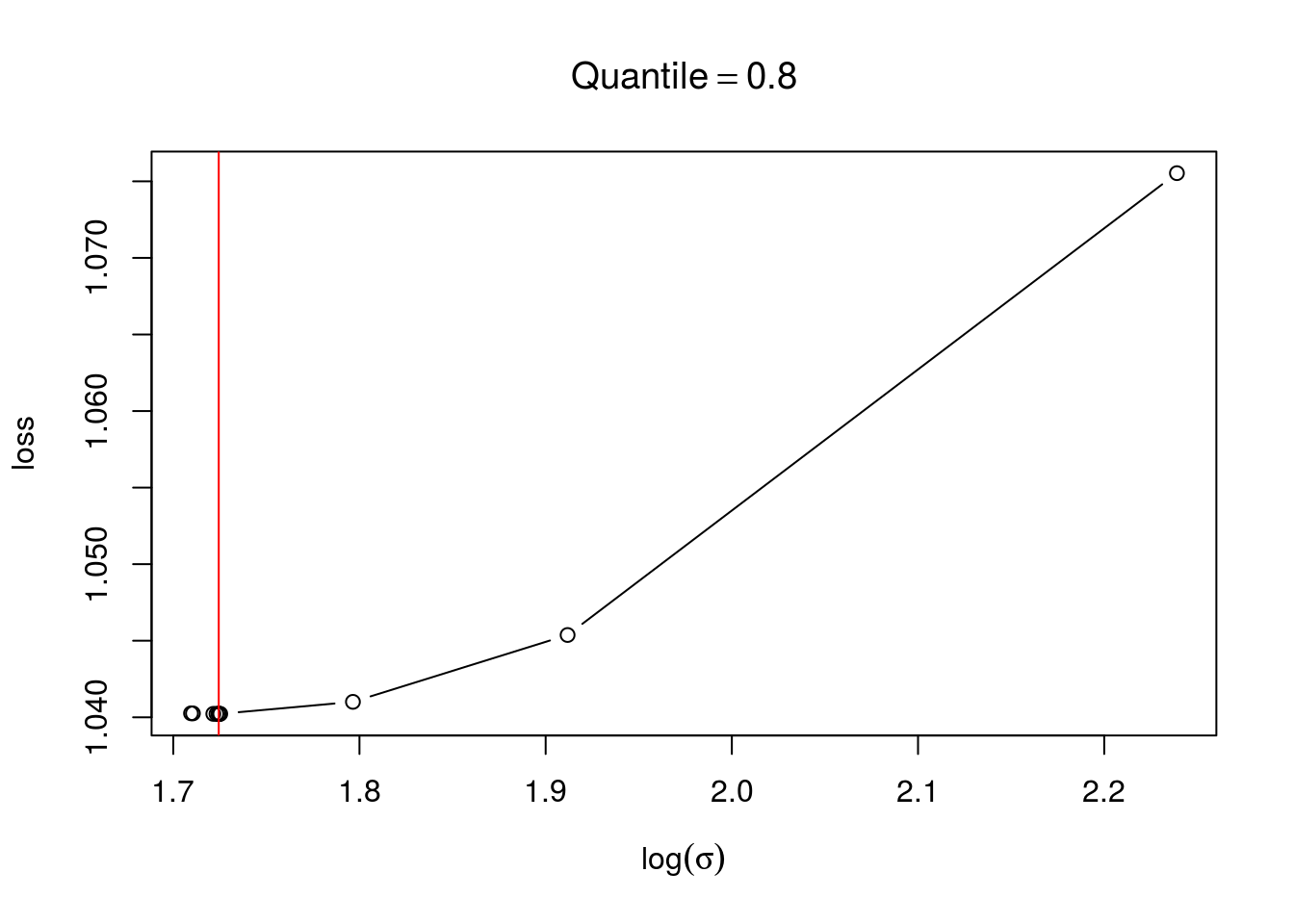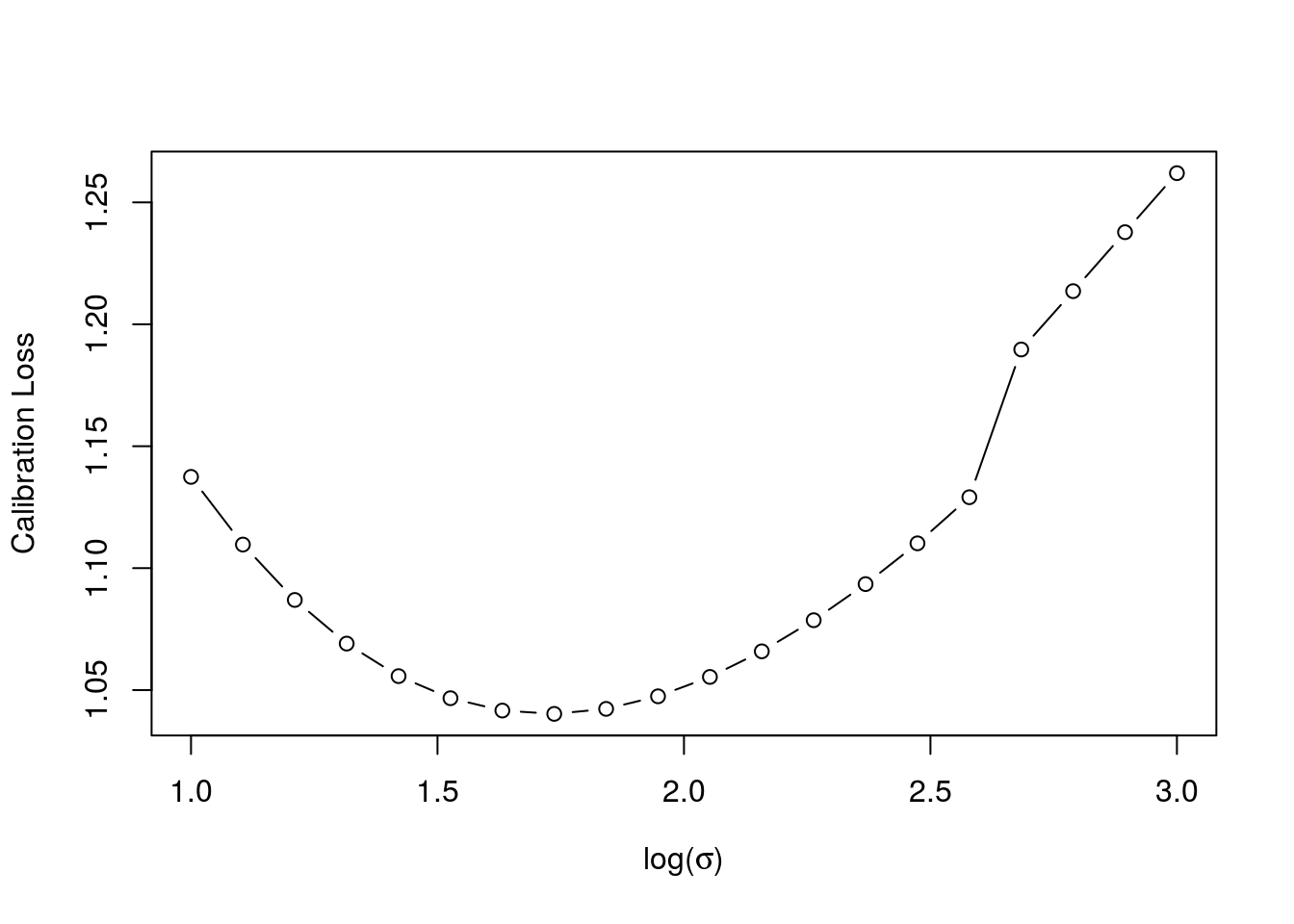This R package offers methods for fitting additive quantile regression models based on splines, using the methods described in Fasiolo et al., 2017.

The main fitting functions are:

• `qgam()` fits an additive quantile regression model to a single quantile. Very similar to `mgcv::gam()`. It returns an object of class `qgam`, which inherits from `mgcv::gamObject`.
• `mqgam()` fits the same additive quantile regression model to several quantiles. It is more efficient that calling `qgam()` several times, especially in terms of memory usage.
• `tuneLearn()` useful for tuning the learning rate of the Gibbs posterior. It evaluates a calibration loss function on a grid of values provided by the user.
• `tuneLearnFast()` similar to `tuneLearn()`, but here the learning rate is selected by minimizing the calibration loss, using Brent method.

# 1 A first example: smoothing the motorcycle dataset

Let’s start with a simple example. Here we are fitting a regression model with an adaptive spline basis to quantile 0.8 of the motorcycle dataset.

``````library(qgam); library(MASS)
if( suppressWarnings(require(RhpcBLASctl)) ){ blas_set_num_threads(1) } # Optional

data = mcycle,
qu = 0.8)``````
``````## Estimating learning rate. Each dot corresponds to a loss evaluation.
## qu = 0.8............done``````
``````# Plot the fit
xSeq <- data.frame(cbind("accel" = rep(0, 1e3), "times" = seq(2, 58, length.out = 1e3)))
pred <- predict(fit, newdata = xSeq, se=TRUE)
plot(mcycle\$times, mcycle\$accel, xlab = "Times", ylab = "Acceleration", ylim = c(-150, 80))
lines(xSeq\$times, pred\$fit, lwd = 1)
lines(xSeq\$times, pred\$fit + 2*pred\$se.fit, lwd = 1, col = 2)
lines(xSeq\$times, pred\$fit - 2*pred\$se.fit, lwd = 1, col = 2)   ```````qgam` automatically calls `tuneLearnFast` to select the learning rate. The results of the calibrations are stored in `fit\$calibr`. We can check whether the optimization succeded as follows:

``check(fit\$calibr, 2)``The plot suggest that the calibration criterion has a single minimum, and that the optimizer has converged to its neighbourhood. Alternatively, we could have selected the learning rate by evaluating the loss function on a grid.

``````set.seed(6436)